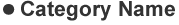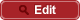• HOME
• RIKEN-QHP
• Measurements of the branching fractions ${\cal B}(B^- \to \bar \Lambda_c^- \Xi_c^0)$, ${\cal B}(B^- \to \bar \Lambda_c^- \Xi_c(2645)^0)$ and ${\cal B}(B^- \to \bar \Lambda_c^- \Xi_c(2790)^0)$

##PrePrint No. RIKEN-QHP-469 Y. Li et al, (Belle Collaboration) (including M. Takizawa) Measurements of the branching fractions ${\cal B}(B^- \to \bar \Lambda_c^- \Xi_c^0)$, ${\cal B}(B^- \to \bar \Lambda_c^- \Xi_c(2645)^0)$ and ${\cal B}(B^- \to \bar \Lambda_c^- \Xi_c(2790)^0)$ arXiv: 1911.12530  (org) Phys. Rev. D 100, 112010 (2019) 2019.11